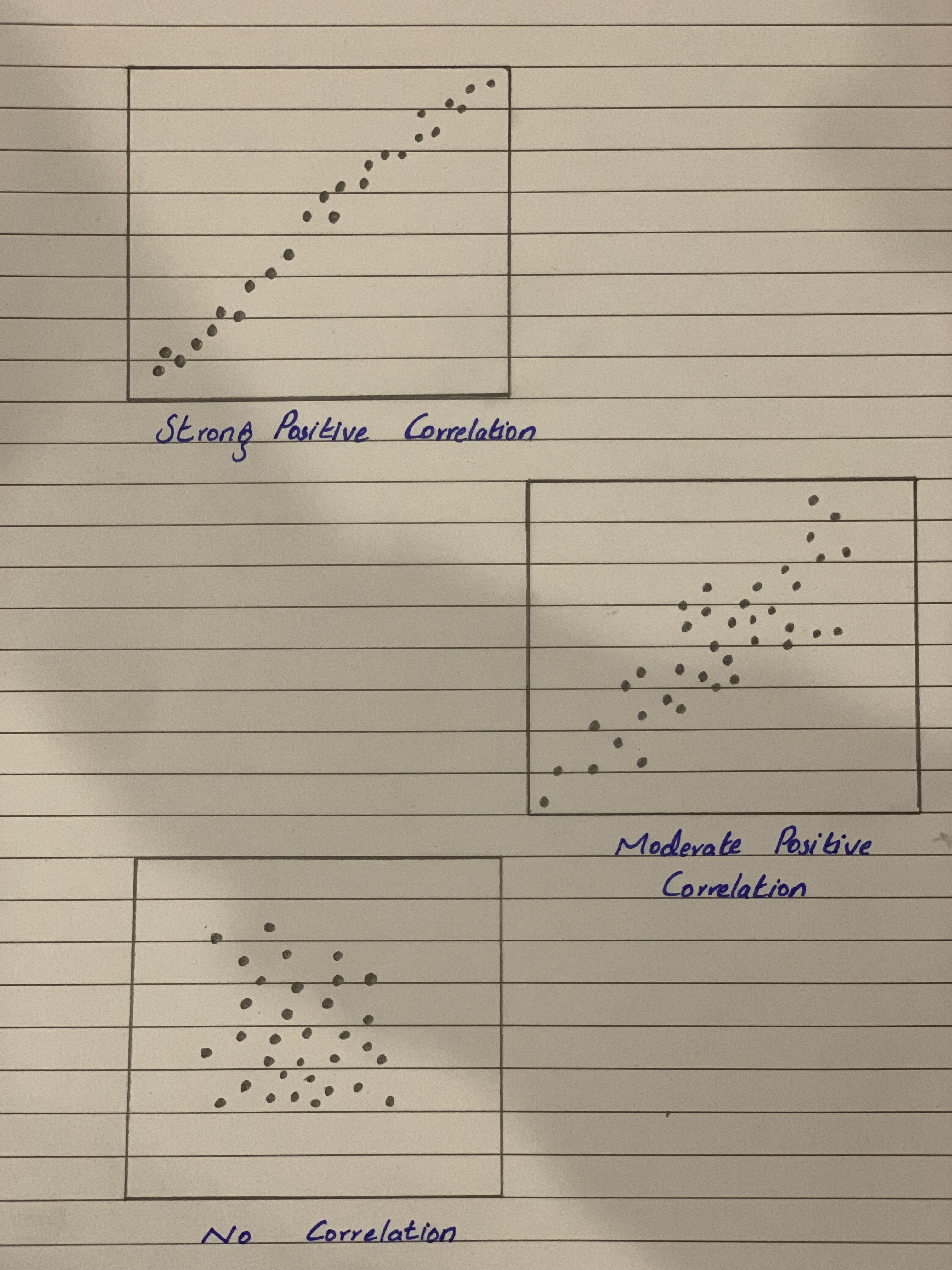# Describe about the three positive relationships of Scatterplots?ka1leE 2021-02-09 Answered
Describe about the three positive relationships of Scatterplots?
You can still ask an expert for help

• Questions are typically answered in as fast as 30 minutes

Solve your problem for the price of one coffee

• Math expert for every subject
• Pay only if we can solve itfirmablogF

Step 1-Introduction
Here's the explanation is as follows,
A scatter plot is a type of graph that shows pairs of data plotted as points.
You can use a scatter plot to analyze trends in your data and to help you to determine whether or not there is a relationship between two variables. A scatter plot can show a positive relationship, a negative relationship, or no relationship.
If the points on the scatter plot seem to form a line that slants up from left to right, there is a positive relationship or positive correlation between the variables.
If the points on the scatter plot seem to form a line that slants down from left to right, there is a negative relationship or negative correlation between the variables.
If the points on the scatter plot seem to be scattered randomly, there is no relationship or no correlation between the variables.
Step 2
Take note that , Scatter plots are similar to line graphs in that they use horizontal and vertical axes to plot data points. However, they have a very specific purpose. Scatter plots show how much one variable is affected by another. The relationship between two variables is called their correlation .
If the data points make a straight line going from the origin out to high x- and y-values, then the variables are said to have a positive correlation .,
Note that ,
Values between 0 and 0.3 indicate a weak positive linear relationship.
Values between 0.3 and 0.7 indicate a moderate positive linear relationship.
Values between 0.7 and 1.0 indicate a strong positive linear relationship